## Monday, March 29, 2010

### Fox 266 - Solutions

Solutions of Fox 266 by Joe, Bleaug, Yu, and Giannno are similar. There is a different geometric solution by Binary Descartes, we should add it in the following days.

Bleaug:
No words needed. (Hey y not? say somethin' :)Yu: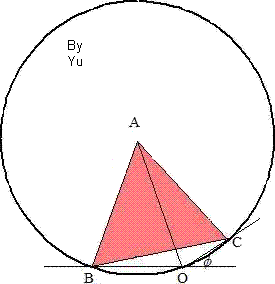Given phi=30°, then m(BOC) = 150° which is 1/2 of reflex m(BAC).
.:B, O and C are on the same circle centred at A. Hence AB = AO.

Giannno:Extend CA such a way that AD=AC. Then m(DBC)=90° (since BA=DC/2 =AD=AC) and m(D)=30° and since m(BOC)=150° we get DBOC cyclic quadrilateral while A is the center of the circle. Hence AB=AO=AC radii of the same circle.
Migue:m(OCB) + m(OBC) = 30°
m(ODA) = 60°
m(DBC) = m(DAC) = 60° - m(BAD) = 60° - m(OCB)
m(DBO) = m(DBC) - m(OBC) = 30°
Triangles DBO and ABO are isosceles. ==> AB = AO.

## Wednesday, March 24, 2010

### Fox 265 - Solutions

Joe has already posted a straightforward solution for Fox 265. Below are several more:

Pure Geometry - Little Trigonometry by Bleaug: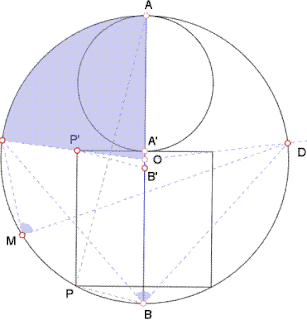a=angle(CMD)=angle(CBD)=angle(AOC)
PP'=AA'=2.A'P' therefore tan(angle(PAB))=1/4. Take B' such that PB//P'B' then angle(A'P'B')=angle(PAB). By construction OA'=OB', so tan(a)=A'P'/OA'=2.A'P'/A'B'=2/tan(angle(A'P'B'))=8.

More Trigonometry by Yu:Radius of the small circle is r, radius of the large circle is R.
tanθ = 1/4, tanΦ=tan2θ=2tanθ/(1-tanθ^2) = 8/15
so, r / sqrt(R^2 - r^2) = 8/15 => R = (17/8)r
tan* = r / (R - 2r) = 8.

Nice use of Pythagoras By Bob Ryden:Inscribed m(ABC) = (1/2) m(AOC) = m(POQ), so we can find m(POQ).
Let M be the midpoint of LN. Draw OM and ON.
Let radius of small circle = a.
Let PO = x.
Then radius of large circle = 2a + x.
OM = 2a – x.
MN = a.
ON = radius of large circle = 2a + x.
OM^2 + MN^2 = ON^2.
(2a – x)^2 + a^2 = (2a + x)^2
a = 8x
tan (ABC) = tan (POQ) = a/x = 8.

http://www.8foxes.com/

## Monday, March 22, 2010

### Fox 267http://www.8foxes.com/
Note that the above is not the only configuration. This one can be classified as "intersection point of the lines can NEVER be in the interior of the equilateral triangle."
Thank you Lou for this nice problem.

## Friday, March 19, 2010

### Fox 266

This simple start will lead to a good one submitted by Lou.

## Thursday, March 18, 2010

### Fox 260 - Solutions

Almost Pure Geometric Solution (An Essential Property of a Parabola)
by Bleaug

Let's take as a parabola "well known" property that for any two points U, V of abscissa u, v, the tangent to point T of abscissa (u+v)/2 is parallel to vector UV. (OK! this demonstration would need some cartesian algebra but simple, promise!) From this we derive that for any point M between U and V the area of triangle UMV et less or equal to area of triangle ATV which maximizes triangle height (e.g. assume the opposite and compare area of triangle obtained from M+dM)Then let's assume U and V achieve the maximum trapezoid area between A (x=0) and B(x=2), then necessarily Au=uv and uv=vB which implies Au=uv=vB=AB/3=2/3. Because of symmetry, maximum area is equivalent to area of rectangle AvVW = 8/9 * 4/3 = 32/27.Geometric Translation
by Yu

Translate y=2x-x^2 to the left by 1 unit to obtain y=1-x^2.Without going into details, the area of the trapezium is greater than the area of the quadrilateral. Area of trapezium, A = (1/2) (2x+2)(1-x^2) = (x+1)(1-x^2)
Max A = 32/27 when x = 1/3.

For more details see Fox 260.
http://www.8foxes.com/

## Wednesday, March 17, 2010

### Fox 265

Polar fox: A little change! Get out of the routine - that's cool, aint it?
Red fox: Little changes lead nowhere! What we need is a whole scale revolution.
Polar fox: What are you suggesting? Rewriting Euclid's axioms?
Red fox: Why not? Define a new basis, set up a new geometry and build a new universe.
Polar fox: Dude, you're beyond here. I think you need a change.

## Sunday, March 14, 2010

### Fox 261 - Solutions

Four good solutions for Fox 261, all drawing lines in the sand...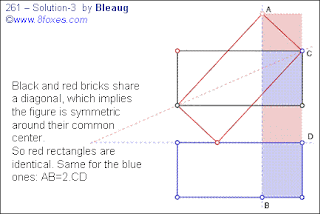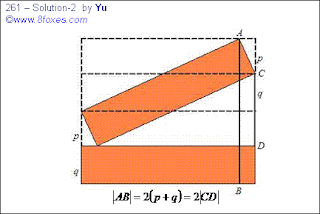http://www.8foxes.com/

## Friday, March 12, 2010

### Fox 263

One more before releasing a general case.

## Tuesday, March 9, 2010

### Fox 261

The claim below makes some information in Fox 252 redundant. This is an intriguing construction! We can easily post half a dozen more good foxes. Keep ckeckin' !!
Also there are at least 3 purely-geometric solutions...

## Saturday, March 6, 2010

### Fox 12

Yu sent a confirmed solution.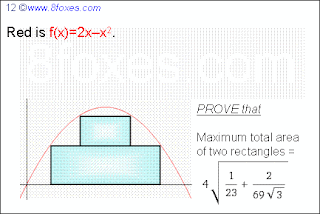http://www.8foxes.com/

## Friday, March 5, 2010

### Fox 11

An old fox resurrects since a new solution has been received.http://www.8foxes.com/

### Fox 255 - Solutions

Median Formula by Wilson: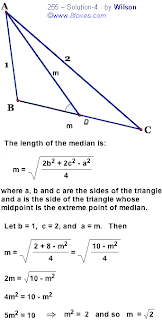Heron's Formula by Wilson: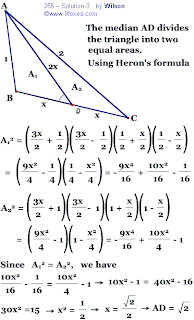Two solutions using cosine rule by Yu:## Thursday, March 4, 2010

### Fox 259

An extension of what Shelby submitted before. Enjoy...
We have not checked if the triangle was constructable. Can it exist with the given lengths?

## Wednesday, March 3, 2010

### Fox 258

Bleaug submitted this as the general case of a series of foxes.http://www.8foxes.com/

## Tuesday, March 2, 2010

### Fox 256 Solution

Submitted by Takyu:Area of kite = 2 × area of triangle AFG
= area of parallelogram ABEF = area of parallelogram ABCD

http://www.8foxes.com/